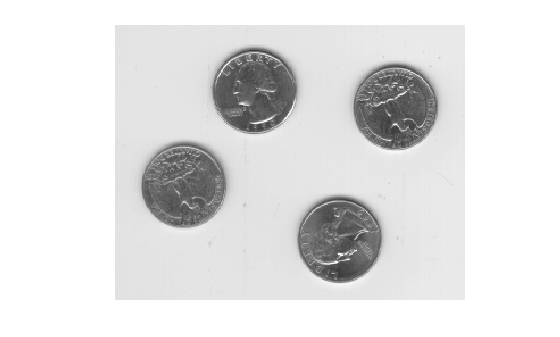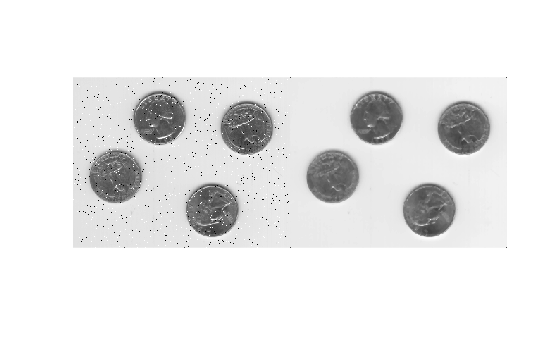Documentation

# medfilt2

2-D median filtering

## Syntax

``J = medfilt2(I)``
``````J = medfilt2(I,[m n])``````
``J = medfilt2(___,padopt)``

## Description

example

````J = medfilt2(I)` performs median filtering of the image `I` in two dimensions. Each output pixel contains the median value in a 3-by-3 neighborhood around the corresponding pixel in the input image.You optionally can compute the normalized cross-correlation using a GPU (requires Parallel Computing Toolbox™).```
``````J = medfilt2(I,[m n])``` performs median filtering, where each output pixel contains the median value in the `m`-by-`n` neighborhood around the corresponding pixel in the input image.```
````J = medfilt2(___,padopt)` controls how `medfilt2` pads the image boundaries.This syntax is not supported on a GPU.```

## Examples

collapse all

Read image into workspace and display it.

```I = imread('eight.tif'); figure, imshow(I)````J = imnoise(I,'salt & pepper',0.02);`

Use a median filter to filter out the noise.

`K = medfilt2(J);`

Display results, side-by-side.

`imshowpair(J,K,'montage')`## Input Arguments

collapse all

Input image, specified as a 2-D grayscale or binary image.

Data Types: `single` | `double` | `int8` | `int16` | `int32` | `uint8` | `uint16` | `uint32` | `logical`

Neighborhood size, specified as a 2-element vector of real positive integers.

If you perform the median filtering using a GPU, then the neighborhood must be square with odd-length sides between 3 and 15.

Data Types: `single` | `double` | `int8` | `int16` | `int32` | `int64` | `uint8` | `uint16` | `uint32` | `uint64`

Padding option, specified as one of the following values.

ValueDescription
`'zeros'` (default)Pad the image with `0`s.
`'symmetric'`Symmetrically extend the image at the boundaries.
`'indexed'`If the class of `I` is `double`, then pad the image with `1`s; otherwise, pad with `0`s.

Data Types: `char` | `string`

## Output Arguments

collapse all

Output image, returned as a numeric matrix of the same class as the input image `I`.

## Tips

• Median filtering is a nonlinear operation often used in image processing to reduce "salt and pepper" noise. A median filter is more effective than convolution when the goal is to simultaneously reduce noise and preserve edges. For information about performance considerations, see `ordfilt2`.

• If the input image `I` is of an integer class, then all the output values are returned as integers. If the number of pixels in the neighborhood (i.e., `m*n`) is even, then some of the median values might not be integers. In these cases, the fractional parts are discarded. Logical input is treated similarly. For example, the true median for the following 2-by-2 neighborhood in a `uint8` array is 4.5, but `medfilt2` discards the fractional part and returns 4.

```1 5 4 8```
• If you specify `padopt` as `'zeros'` or `'indexed'`, then the padding can skew the median near the image boundary. Pixels within one-half the width of the neighborhood (`[m n]/2`) of the edges can appear distorted.

## Algorithms

On the CPU, `medfilt2` uses `ordfilt2` to perform the filtering.

 Lim, Jae S., Two-Dimensional Signal and Image Processing, Englewood Cliffs, NJ, Prentice Hall, 1990, pp. 469-476.